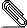```Today i wrote some sample code after a Logic lecture at my university.
The idea is to represent the AST of propositional logic as an ADT with
some convenience functions (like read-/show instances) and then later
perhaps try to make some automatic transformations on the AST.
After construction of the Show instances i found the output a bit boring
and thought that some Unicode math symbols would spice things up. What
happens can be seen in the attached picture (only 3k, that's ok right?).
My terminal supports UTF-8 (when i do cat Logic.hs i can see the unicode
symbols properly).
What might be the problem?```
```
regards
Mattias
```
```module Logic where

infixr 5 :->:, :/\:, :\/:
infixr 5 -->, /\, \/
infix 4 :|-:, :|=:
infix 4 |-, |=

data Logic = Formulae :|-: Formulae
| Formulae :|=: Formulae

data Formulae = Formulae :->: Formulae
| Formulae :/\: Formulae
| Formulae :\/: Formulae
| Formulae :<->: Formulae
| Not Formulae
| P
| Q
| R
| S

-- Operators
(-->) = (:->:)
(/\)  = (:/\:)
(\/)  = (:\/:)
(<->) = (:<->:)
(|-)  = (:|-:)
(|=)  = (:|=:)
--a <-> b = (a --> b) /\ (b --> a)

-- Show

instance Show Logic where
showsPrec d (f1 :|-: f2) = shows f1 . showString " ⊢ " . shows f2
showsPrec d (f1 :|=: f2) = shows f1 . showString " ⊨ " . shows f2

p  = 5
p' = 6
instance Show Formulae where
showsPrec d (f1 :->: f2)  = showBinaryF d f1 " → " f2
showsPrec d (f1 :/\: f2)  = showBinaryF d f1 " ⋀ " f2
showsPrec d (f1 :\/: f2)  = showBinaryF d f1 " ⋁ " f2
showsPrec d (f1 :<->: f2) = showBinaryF d f1 " ↔ " f2
showsPrec d (Not f) = showString "¬"
. showsPrec p' f
showsPrec _ P = showString "p"
showsPrec _ Q = showString "q"
showsPrec _ R = showString "r"
showsPrec _ S = showString "s"

showBinaryF d f1 op f2 = showParen (d > p)
\$ showsPrec p' f1
. showString op
. showsPrec p' f2

```
```import Logic
main = do print (P --> Q |- (Not P) --> (Not Q))```signature.asc
Description: This is a digitally signed message part

```_______________________________________________